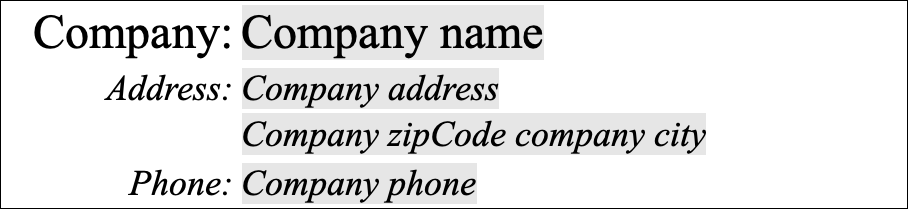# 4D Write Pro: Assign Names to Formulas

In 4D Write Pro documents, formulas are crucial and contribute significantly to their functionality. Previously, these formulas could be represented as values, expressions, or symbols.

However, to further enhance the user experience and improve readability, an exciting new feature with 4D v20 R3 has been introduced: the ability to assign names to formulas. This latest enhancement allows users to quickly identify and comprehend the purpose of each formula within the document.

## FOR WHAT PURPOSE?

Thanks to this new feature, you can display this:## How?

Two commands have been revised and improved.

### WP Insert formula

The first one, the WP Insert formula, now admits two distinct syntaxes.

The first syntax is, of course, retained for the purpose of compatibility:

``````\$formula:=This.data.customer.lastname+" "+This.data.customer.firstname
WP INSERT FORMULA(\$range; \$formula; wk replace)``````

The new one allows you to use an object containing the formula and its name.

``````\$o:=New object
\$o.formula:=Formula(This.data.customer.lastname+" "+This.data.customer.firstname)
\$o.name:="Customer name"
WP INSERT FORMULA(\$range; \$o; wk replace)``````

### WP get formulas

The WP Get formula command has not changed its syntax. It still returns a collection of objects, each corresponding to a formula. These objects have been enriched and now contain the formula name (if defined), the formula itself, and the other existing attributes (range and owner).

``Form.formulas:=WP Get formulas(WParea1)``